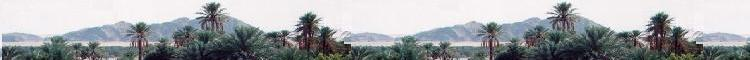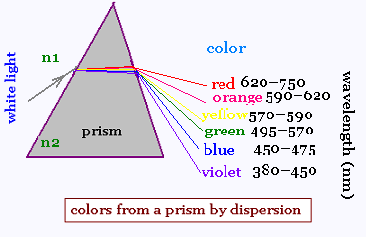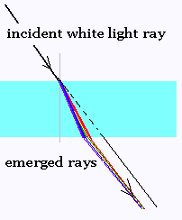##### Optics

Contents

ScientificSentence

# Optics: Index of refraction and Dispersion

The frequency ν of an electromagnetic radiation is given by its source. Its wavelength λ is related to the medium where it propagates. The speed of the radiation v is the product of ν and λ:
v = ν x λ.

### 1. Across the vacuum:

A ray of white light has a speed vo = c = 3.0 x 108 m/s in the vacuum. This constant speed is the commun speed for all its color components ranging from violet to red. This set of colors called optical spectrum. Each of its component has its frenquency , then its wavelength, since λ0 = c/ν. In the vacuum, their wavelengths have the following values in nm (nanometers):

 violet 380�450 blue 450�475 green 495�570 yellow 570�590 orange 590�620 red 620�750

### 2. Across another medium:

The same white ray will have the speed vn in another medium. The frequencies of its spectrum doe not change, because ν is fixed by the source, its wavelengths do, so do their speeds vn = λ0 x μ. Threfore, if a light ray has a speed "c" in the vacuum, its speed becomes vn in a certain medium.

The ratio c/vn = n is called index of refraction of the medium witin the ray passes. In other words, a medium changes the wavelength of a ray, then its speed. For example the green ray has the wavelength range: 495-570 nm, and the speed "c" in the vacuum. In another medium as the Crystal quartz, the wavelength range becomes 315-363 nm and the speed becomes v = 2.0 x 108 m/s approximately. We have then,

for each optical medium, a relationship betweenthe its index of refraction and the wavelength of the traveleing ray.

An index of refraction is not an intrinsic parameter of a medium. For a given optical medium "OP", its index of refraction "n" is function of the wavelength of the traveling ray: nOP = f(λ). This property is called dispersion.

The approximate values of the wavelengths and speeds and given by the well used relationships:

λn = λo/n     vn = c/nwhere "n" is the approximate value of the index of refraction of a the related medium.

Remark:
We can also consider the wavelength of a ray depends on the medium where it propagates and write: λOP = f(n). In literature tables and graphs give the values of n and λ for various substances. The given values of an index of refraction are approximate.The dispersion property explains why a white light ray is broken up into its constituent spectral colors when it enters another medium mainly a prism, as well as a parallal-sided glass slab. For a given prism, each color has its λ then its "n2", then its refracted angle given by Snell's law: r = arcsin[(n1/n2) sin i]. White light is then dispersed.

 chimie labs | Physics and Measurements | Probability & Statistics | Combinatorics - Probability | Chimie | Optics | contact |